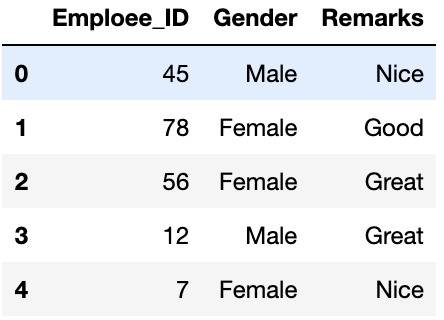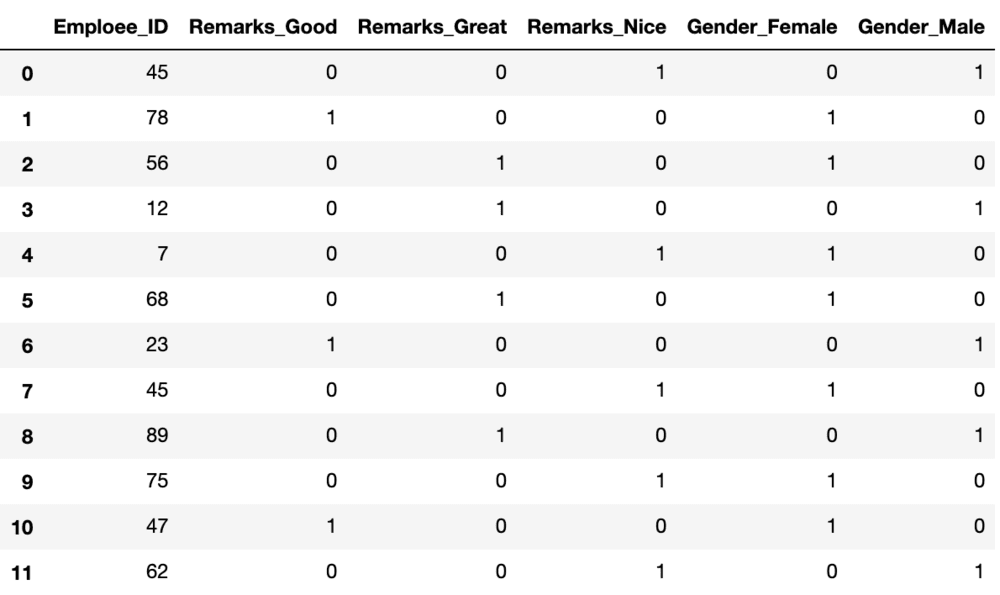GeeksforGeeks App
Open AppBrowser
Continue

# One Hot Encoding in Machine Learning

Most real-life datasets we encounter during our data science project development have columns of mixed data type. These datasets consist of both categorical as well as numerical columns. However, various Machine Learning models do not work with categorical data and to fit this data into the machine learning model it needs to be converted into numerical data. For example, suppose a dataset has a Gender column with categorical elements like Male and  Female. These labels have no specific order of preference and also since the data is string labels, machine learning models misinterpreted that there is some sort of hierarchy in them.

One approach to solve this problem can be label encoding where we will assign a numerical value to these labels for example Male and Female mapped to 0 and 1. But this can add bias in our model as it will start giving higher preference to the Female parameter as 1>0 but ideally, both labels are equally important in the dataset. To deal with this issue we will use the One Hot Encoding technique.

## One Hot Encoding

One hot encoding is a technique that we use to represent categorical variables as numerical values in a machine learning model.

The advantages of using one hot encoding include:

1. It allows the use of categorical variables in models that require numerical input.
2. It can improve model performance by providing more information to the model about the categorical variable.
3. It can help to avoid the problem of ordinality, which can occur when a categorical variable has a natural ordering (e.g. “small”, “medium”, “large”).

#### The disadvantages of using one hot encoding include:

1. It can lead to increased dimensionality, as a separate column is created for each category in the variable. This can make the model more complex and slow to train.

2. It can lead to sparse data, as most observations will have a value of 0 in most of the one-hot encoded columns.

3. It can lead to overfitting, especially if there are many categories in the variable and the sample size is relatively small.

4.  One-hot-encoding is a powerful technique to treat categorical data, but it can lead to increased dimensionality, sparsity, and overfitting. It is important to use it cautiously and consider other methods such as ordinal encoding or binary encoding.

### One Hot Encoding Examples

In One Hot Encoding, the categorical parameters will prepare separate columns for both Male and Female labels. So, wherever there is a Male, the value will be 1 in the Male column and 0 in the Female column, and vice-versa. Let’s understand with an example: Consider the data where fruits, their corresponding categorical values, and prices are given.

The output after applying one-hot encoding on the data is given as follows,

## One-Hot Encoding Using Python

### Creating Dataframe

Creating a dataframe to implement one hot encoding from CSV file.

## Python3

 `# Program for demonstration of one hot encoding`` ` `# import libraries``import` `numpy as np``import` `pandas as pd`` ` `# import the data required``data ``=` `pd.read_csv(``'employee_data.csv'``)``print``(data.head())`

Output:First five rows of Dataframe

### Unique Elements in Categorical Column

we can use the unique() function from the pandas library to get unique elements from the column of the dataframe.

## Python3

 `print``(data[``'Gender'``].unique())``print``(data[``'Remarks'``].unique())`

Output:

```array(['Male', 'Female'], dtype=object)
array(['Nice', 'Good', 'Great'], dtype=object)```

### Count of Elements in the Column

We can use value_counts() function from pandas to get the counts of each element in the dataframe.

## Python3

 `data[``'Gender'``].value_counts()``data[``'Remarks'``].value_counts()`

Output:

```Female    7
Male      5
Name: Gender, dtype: int64

Nice     5
Great    4
Good     3
Name: Remarks, dtype: int64```

We have two methods available to us for performing one-hot encoding on the categorical column.

### One-Hot Encoding of Categorical Column Using Pandas library

We can use pd.get_dummies() function from pandas to one-hot encode the categorical columns. This Function

## Python3

 `one_hot_encoded_data ``=` `pd.get_dummies(data, columns ``=` `[``'Remarks'``, ``'Gender'``])``print``(one_hot_encoded_data)`

Output:One-Hot encoded columns of the dataset

We can observe that we have 3 Remarks and 2 Gender columns in the data. However, you can just use n-1 columns to define parameters if it has n unique labels. For example, if we only keep the Gender_Female column and drop the Gender_Male column, then also we can convey the entire information as when the label is 1, it means female and when the label is 0 it means male. This way we can encode the categorical data and reduce the number of parameters as well.

### One Hot Encoding using Sci-kit Learn Library

Scikit-learn(sklearn) is a popular machine-learning library in Python that provide numerous tools for data preprocessing. It provides a OneHotEncoder function that we use for encoding categorical and numerical variables into binary vectors, also before implementing this algorithm. Make sure the categorical values must are labeled and encoded as one-hot encoding takes only numerical categorical values.

## Python3

 `# importing libraries``import` `pandas as pd``import` `numpy as np``from` `sklearn.preprocessing ``import` `OneHotEncoder`` ` `# Retrieving data``data ``=` `pd.read_csv(``'Employee_data.csv'``)`` ` `# Converting type of columns to category``data[``'Gender'``] ``=` `data[``'Gender'``].astype(``'category'``)``data[``'Remarks'``] ``=` `data[``'Remarks'``].astype(``'category'``)`` ` ` ` `# Assigning numerical values and storing it in another columns``data[``'Gen_new'``] ``=` `data[``'Gender'``].cat.codes``data[``'Rem_new'``] ``=` `data[``'Remarks'``].cat.codes`` ` ` ` `# Create an instance of One-hot-encoder``enc ``=` `OneHotEncoder()`` ` `# Passing encoded columns`` ` `enc_data ``=` `pd.DataFrame(enc.fit_transform(``    ``data[[``'Gen_new'``, ``'Rem_new'``]]).toarray())`` ` `# Merge with main``New_df ``=` `data.join(enc_data)`` ` `print``(New_df)`

Output

```    Employee_Id  Gender Remarks  Gen_new  Rem_new    0    1    2    3    4
0            45    Male    Nice        1        2  0.0  1.0  0.0  0.0  1.0
1            78  Female    Good        0        0  1.0  0.0  1.0  0.0  0.0
2            56  Female   Great        0        1  1.0  0.0  0.0  1.0  0.0
3            12    Male   Great        1        1  0.0  1.0  0.0  1.0  0.0
4             7  Female    Nice        0        2  1.0  0.0  0.0  0.0  1.0
5            68  Female   Great        0        1  1.0  0.0  0.0  1.0  0.0
6            23    Male    Good        1        0  0.0  1.0  1.0  0.0  0.0
7            45  Female    Nice        0        2  1.0  0.0  0.0  0.0  1.0
8            89    Male   Great        1        1  0.0  1.0  0.0  1.0  0.0
9            75  Female    Nice        0        2  1.0  0.0  0.0  0.0  1.0
10           47  Female    Good        0        0  1.0  0.0  1.0  0.0  0.0
11           62    Male    Nice        1        2  0.0  1.0  0.0  0.0  1.0```

NOTE: Here we have converted the enc.fit_transform() method to an array because the fit_transform method of OneHotEncoder returns SpiPy sparse matrix so converting to an array first enables us to save space when we have a huge number of categorical variables.

My Personal Notes arrow_drop_up